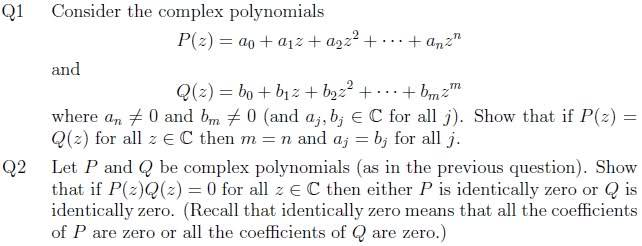# Complex Analysis

Ted123

## Homework Statement## The Attempt at a Solution

How do I go about Q1 and showing the coefficients are unique and then Q2?

Staff Emeritus
Homework Helper
What is your attempt at the solution?

Ted123
What is your attempt at the solution?

I was trying some sort of induction argument.

$P(0)=Q(0) \implies a_0 = b_0$ so the result is true for $z=0$

Now suppose for induction that $P(k)=Q(k)$ ...

Staff Emeritus
Homework Helper
OK, so now take the derivative of the polynomials, and compare $$P^\prime(0)$$ with $$Q^\prime(0)$$.

Ted123
OK, so now take the derivative of the polynomials, and compare $$P^\prime(0)$$ with $$Q^\prime(0)$$.

Bearing in mind z is complex does normal differentiation still hold?

$P'(0)=Q'(0) \implies a_1=b_1$

Last edited:
Ted123
Scrap that method.

Could I say without loss of generality that $n \geq m$ .

Then if $P(z)=Q(z)$ then $P(z)-Q(z)=0$

So collecting up like terms we see that

$P(z) - Q(z) = (a_0 - b_0) + (a_1 - b_1)z + (a_2 - b_2)z^2 + ... + (a_m - b_m)z^m + a_{m+1}z^{m+1} + ... + a_nz^n$

This implies that $a_j=b_j$ for all $0 \leq j \leq m$ (by equating coefficients).

So The first $m$ terms are all 0.

So we have $a_{m+1}z^{m+1} + ... + a_nz^n=0$ .

Can you help me finish?

Ted123
On the other hand saying you can compare coefficients here might be a bit close to begging the question.

Instead, if $P(z) = Q(z)$ for all z and $F(z) = P(z)-Q(z)$, then $1, 2, 3, ..., m+n+1$ are all roots of $F(z)$, and so $(z-1)(z-2)...(z-(m+n+1))$ divides $F(z)$ (factor theorem). Then what does this implies about the degree of F?